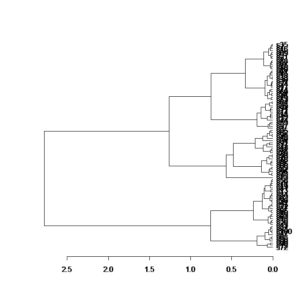# 使用管道操作符%>>%简化R指令行

``` library(vegan)```

`my_dist<-vegdist(dat,"bray")`

`my_clust<-hclust(my_dist,"ward.D")`

`plot(as.dendrogram(my_clust),horiz=T)`

`library(pipeR)`

`library(vegan)`

`vegdist(dat,"bray")%>>%hclust("ward.D")%>>%as.dendrogram%>>%plot(horiz=T)`

`plot(as.dendrogram(hclust(vegdist(dat,"bray"),"ward.D")),horiz=T)`

`options(defaultPackages=c(getOption("defaultPackages"),"pipeR","vegan"))`This site uses Akismet to reduce spam. Learn how your comment data is processed.